U.S. Department of Transportation
1200 New Jersey Avenue, SE
Washington, DC 20590
202-366-4000

Federal Highway Administration Research and Technology
Coordinating, Developing, and Delivering Highway Transportation Innovations

 REPORT This report is an archived publication and may contain dated technical, contact, and link information
 Publication Number:  FHWA-HRT-16-009    Date:  March 2017
 Publication Number: FHWA-HRT-16-009 Date: March 2017

# Using Falling Weight Deflectometer Data With Mechanistic-Empirical Design and Analysis, Volume I: Final Report

## CHAPTER 5. FWD DATA ANALYSIS AND INTERPRETATION—RIGID PAVEMENTS

### Introduction

FWD testing has been traditionally performed on rigid pavements to assess the condition of the pavement to identify the most effective rehabilitation strategy and to establish inputs for an overlay design. FWD data are very useful in determining structural deficiencies before the point when they manifest as a distress. Although FWD data by itself can be quite useful, it is commonly supplemented with a distress survey and possibly GPR testing or destructive testing. Use of the FWD to assess the condition of a rigid pavement includes testing at the corner of the slab for void detection and adjacent to the form transverse joint to monitor joint performance. The MEPDG does not include a direct input for the presence of voids, but this information should be used to determine whether subsealing or rubblization should be performed before the placement of an overlay. The LTE measured at the joints/cracks is also not a required input for the MEPDG but should still be evaluated to determine whether dowel retrofits are necessary before placing the overlay or determining the potential for reflective cracking if a HMA overlay is applied.

FWD testing is also performed at midpanel to backcalculate the material properties for designing an overlay. Historically, the modulus of subgrade reaction (k-value) has been required to characterize the support condition beneath the slab for rigid pavements. When using the MEPDG to design overlays placed on existing PCC pavements, the pavement structure can be characterized by either the modulus of each layer or the elastic modulus of the slab and the modulus of subgrade reaction.

This chapter discusses the approaches available for backcalculating the material properties of a PCC pavement for designing an overlay, analysis and modeling issues associated with these approaches, recommendations for using these approaches with the MEPDG, and future research needs in the area.

### APPROACHES

Several approaches are available for backcalculating the moduli of the PCC slab, base, subbase, and subgrade, as well as the modulus of subgrade reaction. The two primary approaches are a function of how the pavement structure is modeled. The first approach, based on the principles of elastic layered analysis, is typically used for flexible pavements but has also been applied to rigid pavements. The second approach, in which the pavement structure is modeled as either a slab on an elastic solid or on a dense liquid foundation, was specifically developed for rigid pavements based on plate theory.

When the layer moduli are used to define the characteristics of the pavement structure in the MEPDG, the software uses an internal conversion process that automatically determines the effective k-value corresponding to the layer moduli. (As a side note, traditionally, an effective k‑value refers to a k-value that is adjusted for seasonal effects, and the composite k-value refers to the composite stiffness of all layers beneath the slab. The MEPDG documentation refers to an effective k-value as being the composite stiffness of all layers beneath the base, as shown in figure 80.(7)) The process involves backcalculating the effective k-value from the theoretical deflection basin produced using the elastic layer program JULEA. However, in this process, the subgrade resilient modulus is adjusted to reflect the lower deviator stress under PCC pavements (compared with that used in laboratory resilient modulus testing) before generating the deflection basin. Therefore, the subgrade resilient modulus backcalculated from FWD testing cannot be used directly in the MEPDG for PCC or composite pavements because the backcalculated moduli values would reflect the state of stress under PCC pavements, not laboratory testing conditions, which is expected in the MEPDG. Although adjustments could again be made to obtain “laboratory” resilient moduli, the k-value directly backcalculated from the FWD data best represents the true foundation stiffness.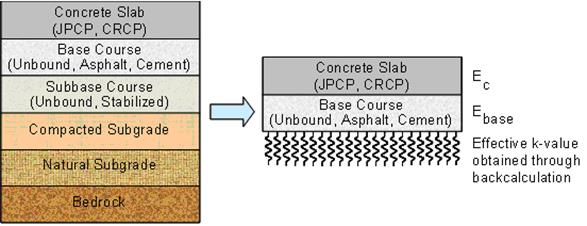JPCP = Jointed portland cement concrete pavement
CRCP = Continuous reinforced concrete pavement

Figure 80. Illustration. E-to-k conversion process incorporated in the MEPDG.(6)

Because the linear elastic layered analysis approach was already discussed in chapter 4 and the preferred input is actually the effective k-value, the focus here is on the methods that are based on the plate theory approach: the Best-Fit and AREA methods. The Best-Fit method minimizes the difference between the predicted and measured deflections at each sensor by solving for the best combination of the radius of relative stiffness and the modulus of subgrade reaction. The AREA method estimates the radius of relative stiffness based on the AREA of the deflection basin. Closed form equations along with the estimated radius of relative stiffness can then be used to estimate the modulus of subgrade reaction and the elastic modulus of the slab. Both methods are based on Westergaard’s solution for interior loading of a linear elastic, homogenous, isotropic plate resting on a dense liquid foundation.

### Available Programs

The two backcalculation procedures based on plate theory (the AREA method and the Best-Fit method) are described in the following sections.

### AREA Method

Hoffman and Thompson first introduced the AREA parameter to characterize the deflection basin for a simple two-parameter backcalculation procedure for flexible pavements.(50) Foxworthy and Darter were the first to apply the AREA concept to the backcalculation for PCC pavements.(92,93) Others refined this approach through the development of efficient, closed-form solutions that replaced the graphical procedure developed by Foxworthy and Darter.(94,49,95) This method has been used quite extensively, in part, because it is the procedure adopted in the 1993 AASHTO Guide for Design Pavement Structures.(32)

The AREA method is based on the unique relationship between the AREA parameter and the radius of relative stiffness, ℓ, which is defined by the equation in figure 81.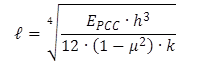Figure 81. Equation. Computation of radius of relative stiffness.

Where:

ℓ = Radius of relative stiffness, mm (inches).
EPCC = PCC elastic modulus, MPa (lbf/inch2).
h = Slab thickness, mm (inches).
μ = PCC Poisson’s ratio.
k = Modulus of subgrade reaction, MPa/mm (lbf/inch2/inch).

The AREA parameter is calculated based on the trapezoidal rule. It is not the actual area under the deflection profile but rather an area normalized with respect to one of the measured deflections. Therefore, AREA has the unit of length and not area. The normalization helps reduce the effects of load magnitude. The AREA definitions developed for three common sensor configurations are provided in the equations in figure 82 through figure 84.(95)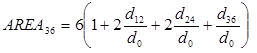Figure 82. Equation. AREA parameter for four-sensor configuration.(95)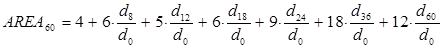Figure 83. Equation. AREA parameter for seven-sensor configuration.(95)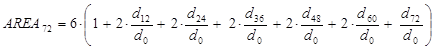Figure 84. Equation. AREA parameter for eight- to nine-sensor configuration.(95)

The relationship between AREA and ℓ is given by the equation in figure 85, with the regression coefficients provided in table 5.(95) These coefficients were defined based on AREA parameters provided in U.S. customary units.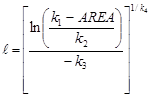Figure 85. Equation. Relationship between AREA and ℓ.(95)

Table 5. Regression coefficients for AREA versus radius of stiffness relationship.(51)

AREA Parameter

k1

k2

k3

1/k4

36

1,812.597

2.559

4.387

60

289.708

0.698

2.566

###### AREA72

72

242.385

0.442

2.205

Note: Coefficients are for use with U.S. customary units.

The radius of relative stiffness is also related to a nondimensional deflection coefficient (d*). Once the radius of relative stiffness is determined, the nondimensional deflection coefficient for any sensor can be determined using the equation in figure 86 and the regression coefficients given in table 6.(95)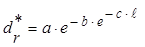Figure 86. Equation. Nondimensional deflection coefficient.(95)

Where:

dr* = Nondimensional deflection coefficient for deflection at radial distance r from load.
a, b, c = Regression coefficients (see table 6).
= Radius of relative stiffness, mm (inches).

Table 6. Regression coefficients for nondimensional deflection coefficient.(51)

Nondimensional
Deflection
Coefficient

a

b

c

d0*

0.12450

0.14707

0.07565

d8*

0.12323

0.46911

0.07209

d12*

0.12188

0.79432

0.07074

d18*

0.11933

1.38363

0.06909

d24*

0.11634

2.06115

0.06775

d36*

0.10960

3.62187

0.06568

d48*

0.10241

5.41549

0.06402

d60*

0.09521

7.41241

0.06255

d72*

0.08822

9.59399

0.06118

Note: Coefficients are for use with U.S. customary units.

These values can then be used to calculate the k-value based on any sensor deflection using the equation in figure 87.(95)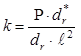Figure 87. Equation. Calculation of k-value.(95)

Where:

k = Modulus of subgrade reaction, MPa/mm (lbf/inch2/inch).
P = Applied load, N (lbf).
dr* = Nondimensional deflection coefficient for deflection at radial distance r from the load.
dr = Measured deflection at radial distance r from the load, mm (inches).
ℓ = Radius of relative stiffness, mm (inches).

The subgrade k-value is then used to determine the PCC modulus by rearranging the equation in figure 81 into the equation shown in figure 88.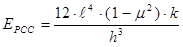Figure 88. Equation. Computation of PCC elastic modulus.

Where:

EPCC = Elastic modulus of the slab, MPa (lbf/inch2).
ℓ = Radius of relative stiffness, mm (inches).
μ = PCC Poisson’s ratio.
k = Modulus of subgrade reaction, MPa/mm (lbf/inch2/inches).
h = Slab thickness, mm (inches).

The subgrade k-value and EPCC for the entire basin can be determined by averaging the values calculated for each deflection sensor.

### Best-Fit Method

The second backcalculation procedure is the Best-Fit method. With this method, the PCC elastic modulus and subgrade k-value are found by identifying the best combination of the two parameters that produces a calculated deflection profile that best matches the measured profile.(51) This is performed through the minimization of the error function provided in figure 89.Figure 89. Equation. Minimization of the error function.

Where:

E = Elastic modulus of the slab, MPa (lbf/inch2).
k = Modulus of subgrade reaction, MPa/mm (lbf/inch²/in).
αi = Weighting factor for deflection measured by sensor i.
wi = Calculated deflection at sensor locatio n i, mm (inches).
Wi = Measured deflection at sensor location i, mm (inches).
n = number of sensors.

The calculated deflection (wi) is based on Westergaard’s solution for interior loading of a plate consisting of a linear elastic, homogenous, and isotropic material on a dense liquid foundation. The weighting factor αi can be set to 1, (1/Wi)2, or any other number as a means of providing flexibility to the Best-Fit solution process.(27) Deflection (w) for sensor i located a distance r from a uniformly distributed circular load can be determined using the equation in figure 90.(96,27)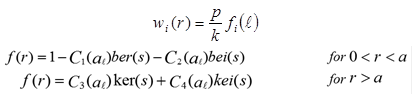Figure 90. Equation. Calculated deflection at specified location.(96,27)

Where:

wi = Calculated deflection at sensor location i, mm (inches).
r = Radial distance of sensor i from the applied load, mm (inches).
k = Modulus of subgrade reaction, MPa/mm (lbf/inch²/inch).
fi (ℓ) = A function of ℓ, distance from the load, and parameters of applied load.
ℓ = (D/k)0.25 Radius of relative stiffness, mm (inches).
s = (r/ℓ) = Normalized radial distance.
ℓ = (D/k)0.25 = Radius of relative stiffness of plate-subgrade system for dense-liquid foundation.
D = Eh3/12(1 – μ2) = Flexural rigidity of the plate.
E = Plate elastic modulus.
m = Plate Poisson’s ratio.
h = Plate thickness.
p = Applied load intensity (pressure) = P/(πa2).

Note that ber, bei, ker, and kei are Kelvin Bessel functions that can be evaluated using appropriate series expressions.(95)

Korenev provided the solutions for the constants C1, C2, C3, and C4 shown in figure 91.(97)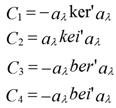Figure 91. Equation. Solutions for C constants.(96)

Where ker', kei', ber', and bei' are the first derivatives of the Kelvin Bessel functions ker, kei, ber, and bei, respectively.

Based on equations in figure 89 and figure 90, the error function can be defined as shown in figure 92.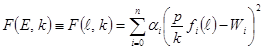Figure 92. Equation. Error function.

The error function is minimized by satisfying the conditions shown in figure 93.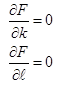Figure 93. Equation. Minimization of error function.

The k-value (figure 94) is determined by substituting the error function equation (figure 92) into the first condition of figure 93.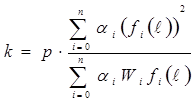Figure 94. Equation. k-value determination.

Substitution of the error function equation (figure 92) into the second condition of figure 93 yields the equation in figure 95 for the radius of relative stiffness.(27)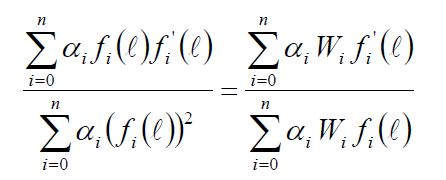Figure 95. Equation. Radius of relative stiffness determination.(27)

The radius of relative stiffness can then be used to determine the elastic modulus of the slab using the equation in figure 88.

The Best-Fit and AREA methods have both been adopted for a pavement structure modeled as a plate on an elastic solid foundation. The procedure was developed based on Losberg’s solution for the distribution of deflections under a load distributed uniformly over a circular area for a plate on an elastic solid.(98) In the case of the Best-Fit method, the minimization of the error function is performed with respect to the elastic modulus of the foundation and the radius of relative stiffness for an elastic solid foundation.(27) It was also found that a unique solution exists between AREA and the elastic solid radius of relative stiffness, making it possible to develop closed-form solutions for the backcalculation of the modulus of an elastic solid foundation using the AREA method.(99) Further details on these methods are not provided because the foundation is not modeled as an elastic solid in the MEPDG.

Both the AREA and Best-Fit methods are relatively easy to use even though software programs are not available for either method. The AREA method has the advantage that it uses simple, closed-form solutions, so a spreadsheet can be easily set up to backcalculate the k-value and the elastic modulus of the slab. A simple computer program can be written to solve for these parameters using the Best-Fit method. The advantage of the Best-Fit method is that backcalculation can be performed for any sensor configuration.

### Base Layer

The methods described are applicable for PCC pavements modeled as slab-on-grade structures. This assumes no structural contribution is provided by the base or subbase layer. In fact, these layers can have a significant effect on the pavement response when the base layer is stiff. This results in an artificially high modulus of the PCC slab. Ioannides and Khazanovich developed an approach for the backcalculation of a two-layered slab-on-grade system.(100) The two layers are modeled as either bonded or unbonded plates, and therefore, these layers are considered incompressible. Both the unbonded and bonded cases are discussed in the following subsections.

Unbonded Case

For the unbonded case, the two plates act independently even though the deflected shapes of the two plates are the same. The effective stiffness of the two plates combined (De) is equal to the sum of the stiffnesses of the upper plate (D1) and the lower plate (D2), as given by the figure 96 equation. De can be determined using the backcalculated elastic modulus from the slab-on-grade analyses presented above using either the AREA or Best-Fit method. The effective stiffness is then determined using the figure 97 equation, which assumes that the thickness of the effective plate, he, is equal to the thickness of the upper plate, h1.(27)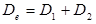Figure 96. Equation. Concept of effective stiffness.(27)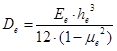Figure 97. Equation. Effective stiffness determination.(27)

The Poisson’s ratio of the effective plate, μe, the upper plate, μ1, and the lower plate, μ2, are assumed to be equal (figure 98) and, therefore, the equation in figure 99 is valid.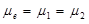Figure 98. Equation. Equivalency of Poisson’s ratio for all plates.(27)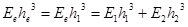Figure 99. Equation. Equivalency of plate stiffnesses.(27)

Where:

E1 = Elastic modulus of the upper plate, MPa (lbf/inch2).
E2 = Elastic modulus of the lower plate, MPa (lbf/inch2).

By introducing an additional input parameter β, the stiffness of the two plates (figure 100) can be determined using the equations in figure 101 and figure 102. β, known as the moduli ratio, is the ratio of the stiffness of the lower plate with respect to that of the upper plate.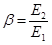Figure 100. Equation. Moduli ratio.(27)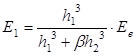Figure 101. Equation. Computation of upper plate elastic modulus.(27)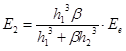Figure 102. Equation. Computation of lower plate elastic modulus.(27)

Bonded Case

When the two plates are bonded, the effective stiffness of the two plates is no longer a summation of the upper and lower plates, but can be defined as shown in figure 103.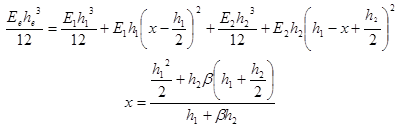Figure 103. Equation. Bonded case effective stiffness.

With the thickness of the effective plate, he, equal to the thickness of the upper plate, h1, the elastic modulus backcalculated based on the slab on-grade pavement structure, Ee, can be used to determine the elastic modulus of the upper plate, E1, as shown in figure 104.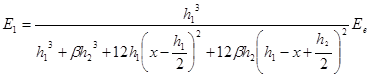Figure 104. Equation. Elastic modulus of the upper plate.

The stiffness of the lower plate, E2, can then be found by multiplying the stiffness of the upper plate by β.

This procedure requires that two pieces of information be known at the time FWD testing is performed: (1) the bond condition between the slab and the base, and (2) the moduli ratio. Engineering judgment is needed in selecting an appropriate moduli ratio. Guidance in selecting a moduli ratio is provided by Khazanovich, Tayabji, and Darter, where a table of typical values for β is provided for a range of base materials.(27)

Fortunately, it has been found that the influence of the moduli ratio on EPCC is not significant for most projects. The modulus of the base is more sensitive to changes in β. The use of an inaccurate moduli ratio that does not affect the backcalculated modulus of the slab could still produce an inaccurate elastic modulus for the base. Again, previous studies have shown that as long as the ratio is within reasonable limits, the results of backcalculation are insensitive to the ratio.(101,27)

As previously noted, the bond condition between the base and the slab must be known to use the two-layered, slab-on-grade analysis. If the slab is assumed to be fully bonded when it actually is unbonded, then unrealistically high moduli will be obtained for the PCC and the base. Likewise, if the slab is assumed to be fully unbonded when it actually is bonded, then unrealistically low moduli will be obtained. The assumed bond condition should be reevaluated and adjusted accordingly if unrealistic moduli are determined.

### Applicability for MEPDG Use

The Best-Fit method was determined to provide more consistent results and has been recommended by other researchers.(51,27) The k-values and slab moduli used to populate the LTPP database were also determined using the Best-Fit method. These values were then used in the development of the MEPDG. The advantage the Best-Fit method has over the AREA method is that the equations can easily be placed in a spreadsheet, making the backcalculation of the moduli very simple.

Another matter to consider when performing backcalculation is defining which sensor configuration will provide the best results based on the backcalculation method to be used. When using the Best-Fit method, it has been found that the four-sensor configuration yielded more reasonable results a greater percentage of the time than the seven-sensor configuration (the seven- and four-sensor configurations are listed in table 7).(51) Therefore, it is recommended that the four-sensor configuration be used for the Best-Fit method.

Table 7. Distances of each sensor from the applied load for the seven- and four-sensor configurations.(51)

Sensor Configuration

Sensor Position (mm)

Seven

0, 203, 457, 610, 914, and 1,524

Four

0, 305, 610, and 914

25.4 mm = 1 inch

The AREA and Best-Fit methods give comparable results, although the AREA method tends to produce k-values that are slightly higher than the values obtained from the Best-Fit method. This is largely because of the compressibility of the PCC slab.(27) However, given that good agreement was found between the Best-Fit method with the four-sensor configuration and the AREA method with the seven-sensor configuration, the latter method is also recommended for use in backcalculating moduli.(51,27)

### Accuracy and Reliability

The reliability of the backcalculation results can be checked by comparing the predicted deflection basin with the measured deflection basin. The relative error between the measured deflection at each sensor and deflections calculated using the backcalculated parameters can be found using the equation in figure 105. The average of the absolute relative errors for all of the sensors is the absolute relative error of the deflection basin (figure 106).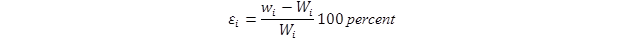Figure 105. Equation. Relative error between the measured and calculated deflections.

Where:

εi = Relative error for sensor i, percent.
wi = Calculated deflection at sensor location i, mm (inches).
Wi = Measured deflection at sensor location i, mm (inches).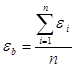Figure 106. Equation. Mean absolute relative error for deflection basin.

Where:

εb = Mean absolute relative error for a deflection basin, percent.
εi = Relative error for sensor i, percent.
n = Number of sensors used to define the deflection basin.

A tolerance level of 2 percent for the mean absolute relative error for the deflection basin is considered acceptable.(27)

A check on the validity of the backcalculation data should include not only a comparison of the measured and calculated deflection basins at each test location but also the variability of the backcalculated parameters along the test section. The coefficient of variation for the backcalculated k should be less than 20 percent along any particular section once reasonable outliers have been removed.(51) The coefficient of variation for the radius of relative stiffness should be less than 10 percent.(27) Factors that might result in coefficients of variation greater than this include changes in subgrade type, depth to bedrock, layer thicknesses, layer conditions, and slab curling conditions.(51,27)

### Analysis and Modeling Issues

Various analysis and modeling issues affect the ability to accurately backcalculate E and k‑values. The most prominent of these issues are discussed in following subsections.

### Temperature Effects

Slab curling and/or warping can significantly influence the deflection response of PCC pavements, but none of the existing methods account for the effects of slab curling.(27,102) Khazanovich, Tayabji, and Darter showed k-values backcalculated at one location to be up to three times as high as a result of temperature gradients.(27) The magnitude of the effect of the gradient is a function of the pavement structure being tested. Vandenbossche found that temperature gradients have a greater influence on backcalculated k-values for thinner slabs compared with thicker slabs because the deflections measured on thicker slabs are less sensitive to changes in support conditions.(102) Currently, there is no method available for correcting for the effects of slab curvature when backcalculating k-values. It is recommended that FWD testing be performed when there is no significant temperature gradient present to avoid any influence slab curling might have on the backcalculated EPCC and k-values.

While temperature and moisture gradients can have an effect on the backcalculated EPCC and k‑values, researchers have found that uniform changes in temperature have little to no effect.(102,103) Only large temperature fluctuations (temperatures outside of 7 to 32 °C (45 to 90°F)) substantially influence the backcalculated EPCC and k-values.(103) In general, changes in the uniform temperature produce very little variation above and beyond what is typically inherent to the equipment and pavement materials.

### Slab Size

The backcalculation procedure presented is based on Westergaard’s solution for an infinite plate, but pavements have a finite length and width. Correction factors have been developed for determining the elastic modulus and k-value for the interior loading of a square slab by Crovetti using the following procedure:(104)

• Step 1: Estimate ℓ (ℓest ) using either the Best-Fit or AREA method.

• Step 2: Calculate L/ est, where L is the length of the sides of the square slab.

• Step 3: Calculate adjustment factors for the deflection directly under the load plate (do) and radius of relative stiffness (ℓ) using equations in figure 107 and figure 108.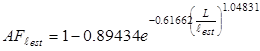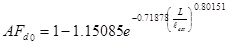Figure 108. Equation. Adjustment factor for the deflection directly under the load plate.(104)

• Step 4: Calculate adjusted d0, where adjusted d0 is the measured d0 × (AFdo).

• Step 5: Calculate adjusted ℓ, where adjusted ℓ = ℓ est × (AF lest).

• Step 6: Backcalculate EPCC and k-value using the adjusted d0 and the adjusted ℓ.

This method was expanded upon to address rectangular slabs by using an L that represents a square slab having the same area as the rectangular slab, as shown in the figure 109 equation.(27)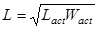Figure 109. Equation. Conversion to equivalent square slab.(27)

Where:

L = Length of square slab, m (ft).
Lact = Actual slab length, m (ft).
Wact = Actual slab width, m (ft).

For longer slabs, where the slab length is greater than twice the width, the equivalent slab length is equal to the value calculated using the equation in figure 110.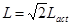Figure 110. Equation. Conversion to equivalent square slab (slab length greater than twice the width).

The k-value is then calculated using the figure 111 equation instead of the adjusted d0.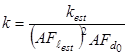Figure 111. Equation. Calculation of k-value.

The k-value and the adjusted ℓ can then be used to determine EPCC using the figure 88 equation.

Corrections for slab length are not frequently used because it is difficult to define the effective length and width of the slab. The effective length and width are a function of the LTE at the adjacent joints. While this information might be available for the adjacent transverse joints, the load transfer across the lane/shoulder and centerline joints are typically not known. Therefore, defining the effective width becomes difficult.

The load magnitude for roadways is typically sufficient that varying load levels does not affect the backcalculation results for PCC pavements. Evidence of this is provided in the study by Khazanovich, Tayabji, and Darter.(27) Larger loads would be necessary for airport pavements.

### Backcalculated Versus Laboratory Modulus

Khazanovich, Tayabji, and Darter compared the backcalculated EPCC with the static chord modulus measured using ASTM C469 for cores pulled from LTPP rigid pavement sections with aggregate bases.(27,105) A very poor correlation was found, which can at least partly be attributed to curling/warping of the slab and the inability of the subgrade models to capture the actual subgrade response. The backcalculated moduli are also substantially greater than the measured static values. This is not surprising because a dynamic modulus is being compared with a static modulus. The difference between a static and dynamic modulus is discussed in more detail in the following section. Part of this discrepancy might also be attributable to the difference in the magnitude of the stress where the stress-strain relationship is being defined. PCC is modeled as a linear elastic material, but it actually is a nonlinear inelastic material in both tension and compression. The magnitude of the backcalculated modulus lies somewhere between the initial tangent modulus obtained using ASTM C469 and the chord modulus because the stress magnitude used to define the initial tangent modulus is substantially lower than that used for the chord modulus.(105)

Although the MEPDG requires a dynamic k-value, the input for PCC modulus is the static chord modulus measured based on ASTM C469.(7,105) The MEPDG recommends multiplying the backcalculated PCC elastic modulus by 0.8 to convert from a backcalculated modulus to a value measured in the laboratory using ASTM C469.(7,105)

### Dynamic Backcalculation

Backcalculation methods often assume a quasi-static pavement response during FWD testing. This assumes the peak deflection at each sensor occurs at the same time as the peak load. What actually happens is that the peak load occurs first, followed by the peak deflections. There is a significant lag in time between the peak load and the peak deflection for the sensor under the load plate and among the peaks of the sensor deflections. The lag can be attributed to the inertia of the pavement system and damping effects.(52)

Some of the seasonal variation in backcalculated moduli can be attributed to the use of static backcalculation procedures for the analysis of a dynamic problem. It has been found that not only the backcalculated k-value, but also the backcalculated PCC moduli, show seasonal variation for pavements in colder climates. The PCC elastic modulus increases when temperatures are colder. This increase is larger than what would typically be expected as a result of the change in the temperature. Part of this increase in moduli can be attributed to an increase in the mass of the plate moved by the FWD load and variation in the subgrade damping due to the effects of the temperature and moisture on the lower layers.(52)

Khazanovich presented a closed-form solution to describe the dynamic behavior of a linear elastic, homogeneous, and isotropic plate on a dense liquid foundation based on Westergaard’s solution for an interior loading.(52)

### RECOMMENDATIONS FOR MEPDG USE

The input parameters needed for the design of an overlay on top of a PCC pavement using the MEPDG that can be extracted from FWD data include the elastic modulus of the existing PCC and base layers, the subgrade k-value, and the PCC flexural strength. The moduli for each layer can also be backcalculated using layer elastic theory as described in chapter 4, but as previously discussed, it is better to define the stiffness of the lower layers with the backcalculated k-value. Recommendations to consider when determining these inputs, based on FWD data, are provided in the following subsections.

### Effective k-Value

As previously discussed, the ideal method for characterizing the subgrade in the MEPDG is by backcalculating the effective k-value, which represents the stiffness of all layers beneath the base. The user will find that the program still requires the moduli of each layer to be entered as well.(7) This is a glitch in the program. Even though the layer moduli are required to be entered, the values entered will not affect the design. The design will only consider the effective k-value. It is important to correctly enter in the other material characterization properties, such as the gradations of these layers, because this information is used along with the Enhanced Integrated Climatic Model (EICM) to estimate the seasonal effects on the k-value. When entering the k‑value, the designer must also enter the month in which the k-value was measured. Seasonal corrections are then applied to the k-value based on the moisture conditions predicted through the EICM. It is assumed that the correction factors for the k-value are the same as the adjustment ratios that would be used for the moisture corrections of the resilient moduli if these values were entered instead of a k-value.

It is important to note that the subgrade k-value determined from backcalculation of FWD data is a dynamic k-value, which may be up to three times higher than a static value.(106) Although the MEPDG requires a dynamic k-value, many design procedures require the use of a static k-value. For instance, in the AASHTO 1993 design procedure, the backcalculated k-value is divided by 2.0 to obtain a static k-value for use in design.(32)

### PCC Elastic Modulus

The elastic modulus of the existing slab must be determined for overlay designs and existing JPCP being considered for restoration. The elastic modulus can be determined by pulling a core and measuring the chord modulus based on ASTM C469 or by using FWD data to backcalculate the modulus.(105)A backcalculated modulus must be multiplied by 0.8 to convert from a dynamic to a static elastic modulus.

For an unbonded overlay, the static elastic modulus of the PCC pavement that is determined using backcalculation or laboratory testing must be adjusted to reflect the overall condition of the pavement. The modulus is adjusted based on the condition of the pavement by multiplying it by the appropriate condition factor. Condition factors for a range of pavement conditions are provided in table 8. The pavement condition is defined based on the accumulated damage. The accumulated damage for a JPCP is a function of the percent slabs cracked (see table 9) and for a CRCP, it is based on the number of punchouts per mile (see table 10). The damage factor can then be used along with table 11 to provide an estimate of the pavement condition.

For restoration, the elastic modulus is assumed not to increase over time because the strength and stiffness of old concrete will not significantly change.

Table 8. Recommended condition factor values used to adjust moduli of intact slabs.(6)

Qualitative Description of
Pavement Condition

Recommended Condition Factor, C

Good

0.42 to 0.75

Moderate

0.22 to 0.42

Severe

0.042 to 0.22

Table 9 through table 11 provide guidelines to assess pavement condition.

Table 9. Damage estimates for JPCP based on percent slabs cracked.(6)

Percent Slabs Cracked

Damage

0

0.100–0.250

10

0.270

20

0.438

30

0.604

40

0.786

50

1.000

Table 10. Damage estimates for CRCP based on punchouts per mile.(6)

Number of Punchouts
Per Mile

Damage

0

0.10–0.15

2

0.22

4

0.34

6

0.44

8

0.53

10

0.62

> 10

> 0.62

1 mile = 1.61 km.

Table 11. Pavement condition rating based on damage estimates for JPCP and CRCP.(6)

Category

Damage

Excellent

0.10–0.25

Good

0.50–0.67

Fair

1.00

Poor

> 1.00

Very Poor

>> 1.00

### PCC Flexural Strength

The PCC flexural strength of the existing slab must also be determined for an overlay design. This is best determined by measuring the split tensile strength of cores and then using the split tensile strength to estimate the flexural strength. These cores can also be used to determine the slab thickness because backcalculation is highly sensitive to layer thicknesses. As a possible level 3 input, Foxworthy’s correlation between the backcalculated modulus of the slab, as presented in the figure 112 equation, can be used to estimate the flexural strength.(103) This relationship was established based on limited data (n = 13) and should therefore be used with caution. The coefficient of determination for this relationship is R2 = 0.71.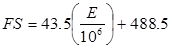Figure 112. Equation. Estimate of flexural strength based on elastic modulus.(103)

Where:

FS = Flexural strength estimated for indirect tensile strength, lb/inch2.
E = In situ modulus of elasticity, backcalculated from FWD data, lb/inch2.

### Future Research Needs

Substantial improvements could be made in the current backcalculation process to help reduce the variability found between backcalculated moduli calculated at the same location at different times. The development of correction factors that account for dynamic effects would help reduce seasonal variability. These correction factors would be used to account for the effects of changes in the inertia of the pavement system and damping of the subgrade. Curling/warping of the slab, on the other hand, can increase the variability of the backcalculated moduli calculated at the same test location throughout the day, although the magnitude of the variability fluctuates seasonally. With the large quantity of rehabilitation work that needs to be performed by many State agencies, it is not feasible to limit FWD testing to those time periods when gradients are not likely to be present. For this reason, the development of correction factors that account for the effects of temperature/moisture gradients in the slab on backcalculated moduli would be very useful. Some steps have already been taken to help address these issues.(52,102,104)

Federal Highway Administration | 1200 New Jersey Avenue, SE | Washington, DC 20590 | 202-366-4000
Turner-Fairbank Highway Research Center | 6300 Georgetown Pike | McLean, VA | 22101